﻿ L_WindowLevelFillLUT - Raster Imaging C API Help
In This Topic ▼

# L_WindowLevelFillLUT

#include "l_bitmap.h"

L_LTDIS_API L_INT L_WindowLevelFillLUT (pLUT, ulLUTLen,crStart, crEnd, nLow, nHigh, uLowBit, uHighBit, nMinValue, nMaxValue, nFactor, uFlags)

 L_RGBQUAD* pLUT; lookup table L_UINT ulLUTLen; number of entries L_COLORREF crStart; start color L_COLORREF crEnd; end color L_INT nLow; low value for leveling L_INT nHigh; high value for leveling L_UINT uLowBit; low bit L_UINT uHighBit; high bit L_INT nMinValue; bitmaps minimum value L_INT nMaxValue; bitmaps maximum value L_INT nFactor; factor L_UINT uFlags; flags

Fills the user-allocated 8-bit LUT with values range between crStart and crEnd colors according to the selected LUT type.

 Parameter Description pLUT Pointer to an array to be updated with the RGB quad (i.e. lookup table). ulLUTLen The size of pLUT. The minimum size is 2 raised to the power of (uHighBit - uLowBit + 1). crStart Starting color value for the gradient. crEnd Ending color value for the gradient. nLow The low value of the window width, in pixels. nHigh The high value for the window width, in pixels. uLowBit Value indicating the low bit used for leveling. This is normally 0 and should be less then the uHighBit. uHighBit Value indicating the high bit used for leveling. This should be greater or equal to uLowBit and less then 11 for 12-bit grayscale or 15 for 16-bit grayscale. nMinValue The bitmap's minimum value. This value can be obtained by L_GetMinMaxVal. nMaxValue The bitmap's maximum value. This value can be obtained by L_GetMinMaxVal. nFactor Value that indicates the factor to be applied in the function operation specified in the uFlag parameter. This parameter is used only if uFlags is FILLLUT_EXPONENTIAL, FILLLUT_LOGARITHMIC or FILLLUT_SIGMOID. If FILLLUT_EXPONENTIAL or FILLLUT_SIGMOID flag is selected its value can be any integer (+/-). If FILLLUT_LOGARITHMIC flag is selected its value should be >= 0. If nFactor = 0, the lookup table will be filled linearly. uFlags Flags that indicate how the range is used to fill the LUT and the type of LUT and whether the LUT contains signed or unsigned data. The following flags indicate how the range is used to fill the LUT: Value Meaning FILLLUT_INSIDE [0x0001] Fill the LUT between nLow and nHigh. ( inside the nLow...nHigh range ) FILLLUT_OUTSIDE [0x0002] Fill the LUT below nLow and above nHigh and also the in between. The following flags indicate the LUT type: Value Meaning FILLLUT_LINEAR [0x0010] LUT is linear. FILLLUT_EXPONENTIAL [0x0020] LUT is exponential. FILLLUT_LOGARITHMIC [0x0030] LUT is logarithmic. FILLLUT_SIGMOID [0x0040] LUT is sigmoid. The following flags indicate whether the LUT contains signed or unsigned data: Value Meaning FILLLUT_UNSIGNED [0x0000] The LUT data is unsigned. FILLLUT_SIGNED [0x0100] The LUT data is signed. The following flag indicates whether to fill the LUT only using crStart and crEnd colors as used in application using DICOM: FILLLUT_DICOM_STYLE [0x1000] Fill using crStart and crEnd colors only

#### Returns

 SUCCESS The function was successful. < 1 An error occurred. Refer to Return Codes.

## Comments

Use this function to fill in the LUT, used in L_WindowLevel or L_WindowLevelBitmap, according to LUT type flag.

This function fills an 8-bit LUT. If you want to fill a 16-bit LUT, see L_WindowLevelFillLUTExt.

The LUT will be filled as follows:

 Inside/Outside nMinValue-nLow nLow-nHigh nHigh-nMaxValue FILLLUT_INSIDE solid black (0,0,0) color gradient ranging from crStart to crEnd solid white (255,255,255) FILLLUT_OUTSIDE solid color (crStart) grayscale values from solid black (0,0,0) to solid white (255, 255, 255) solid color (crEnd) FILLLUT_INSIDE | FILLLUT_DICOM_STYLE none color gradient ranging from crStart to crEnd None FILLLUT_OUTSIDE| FILLLUT_DICOM_STYLE solid color (crStart) color gradient ranging from crStart to crEnd solid color (crEnd)

The nFactor parameter is used for log, exp and sigmoid functions only. If nFactor = 0 the function performs a linear interpolation between the two points nLow and nHigh and stores the results in the lookup table, regardless of the value in uFlags.

If uFlag is FILLLUT_EXPONENTIAL the value of nFactor modifies the lookup table values (see figure below) according to the following equations: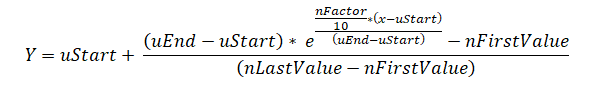where: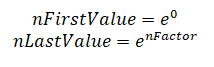x = the intensity value of the selected point

uStart = the nLow parameter of this function

uEnd = the nHigh parameter of this function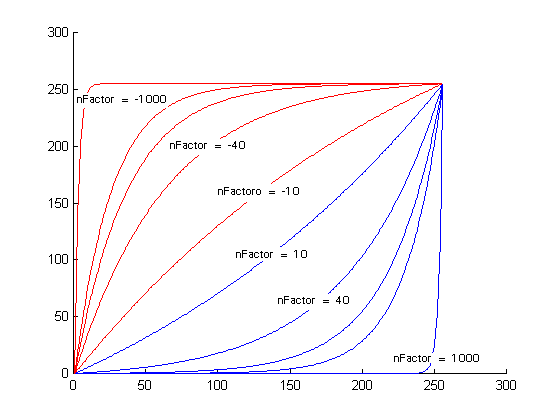If uFlag is FILLLUT_LOGARITHMIC the value of nFactor modifies the lookup table values (see figure below) according to the following equations: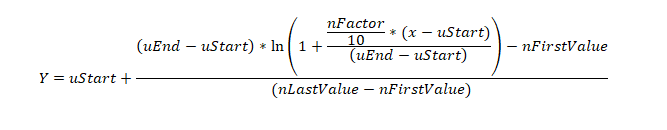where: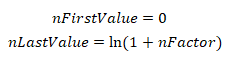x = the intensity value of the selected point

uStart = the nLow parameter of this function

uEnd = the nHigh parameter of this function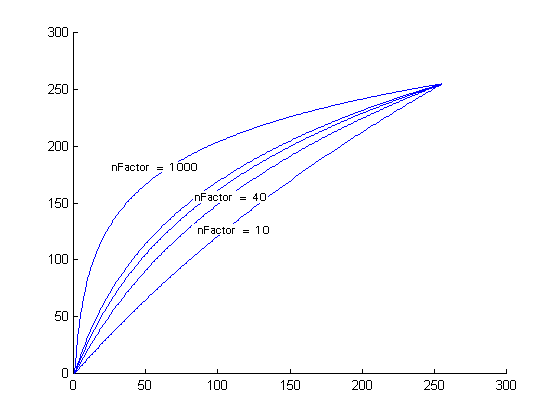If uFlag is FILLLUT_SIGMOID the value of nFactor modifies the lookup table values (see figure below)according to the following equations: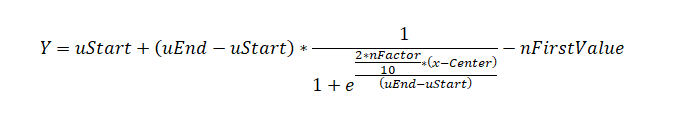where: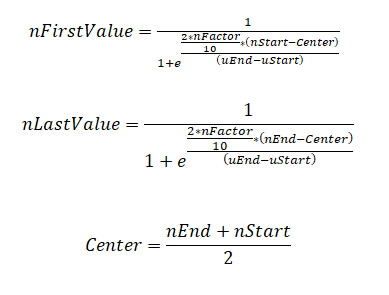x = the intensity value of the selected point

uStart = the nLow parameter of this function

uEnd = the nHigh parameter of this function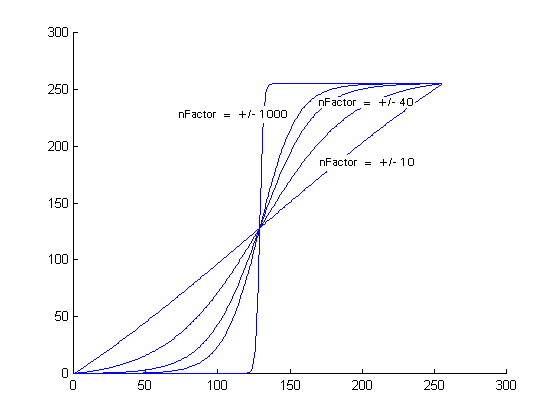If uFlag is FILLLUT_LINEAR, nFactor is ignored. The function fills the lookup table linearly.

Note: This function only works for 12 or 16 bit grayscale images. Trying to use this function with other images will result in an error.

You must allocate the memory for the LUT before calling this function. You can calculate the required size with (sizeof(RGBQUAD) * (1<<(pBitmap->HighBit - pBitmap->LowBit + 1))), as seen in the example below.

For example, suppose you are working with a 12 bit grayscale image. There are 4096 intensity levels in a 12 bit image (2 raised to the 12th power). Normally, the interval between 0 and 4095 for unsigned data would be mapped to colors between (0, 0, 0) and (255, 255, 255). With this function, any value that falls between the low level and the high level will be mapped to colors between the start color and the end color. If you do not want a mapping function, set the start and end colors equal.

If you only want to map the values between 1972 and 3273 (in a 12-bit unsigned image), then set nLow to 1972, set nHigh to 3273, and set FILLLUT_INSIDE option. To map the values less than 1972 and greater than 3273, select FILLLUT_OUTSIDE option.

LEADTOOLS supports two types of LUTs for 10-16-bit grayscale images (8-bit LUT and 16-bit LUT).  Typical grayscale image display and processing is done using an 8-bit LUT.  But, you can also use a 16-bit LUT, which offers more precision.  Some special video cards and monitors also support display of grayscale images using a 16-bit LUT.

For information on saving bitmaps that have been window leveled, refer to Saving Window-Leveled Bitmaps.

Required DLLs and Libraries

 LTDIS For a listing of the exact DLLs and Libraries needed, based on the toolkit version, refer to Files To Be Included With Your Application.

Platforms

Win32, x64, Linux.

See Also

## Example

````L_INT WindowLevelFillLUTExample(pBITMAPHANDLE pBitmap) `
`{ `
`   L_INT nRet; `
`   RGBQUAD* pLUT; `
`   L_INT    nMin, nMax; `
`   L_INT    nLowBit, nHighBit; `
`   L_UINT   uSigned; `
` `
`   /* allocate the lookup table */ `
`   pLUT = (RGBQUAD *)malloc(sizeof(RGBQUAD) * (L_INT32)(1<<(pBitmap->HighBit - pBitmap->LowBit + 1))); `
` `
`   /* get the bitmap's min/max values */ `
`   nRet = L_GetMinMaxBits(pBitmap, &nLowBit, &nHighBit, 0); `
`   if(nRet != SUCCESS) `
`      return nRet; `
`   nRet = L_GetMinMaxVal(pBitmap, &nMin, &nMax, 0); `
`   if(nRet != SUCCESS) `
`      return nRet; `
`    `
`   nLowBit  = pBitmap->LowBit; `
`   nHighBit = pBitmap->HighBit; `
`    `
`   if(pBitmap->Flags.Signed) `
`      uSigned = FILLLUT_SIGNED; `
`   else `
`      uSigned = FILLLUT_UNSIGNED; `
`     `
`   /* fill the LUT with a color gradient */ `
`   nRet = L_WindowLevelFillLUT((RGBQUAD*) pLUT, `
`                               1<<(pBitmap->HighBit - pBitmap->LowBit + 1), `
`                               RGB(255,0,0),    /* RED              */ `
`                               RGB(0,0,255),    /* BLUE             */ `
`                               23000,           /* Starting value   */ `
`                               45000,           /* Ending value     */ `
`                               nLowBit,         /* Bitmap's LowBit  */ `
`                               nHighBit,        /* Bitmap's HighBit */ `
`                               nMin,            /* Bitmap's MinVal  */ `
`                               nMax,            /* Bitmap's MaxVal  */ `
`                               10,              /* nFactor */ `
`                               FILLLUT_INSIDE | FILLLUT_LINEAR | uSigned); /* Fill Inside*/ `
`   if(nRet != SUCCESS) `
`      return nRet; `
` `
` `
`   /* window level the bitmap using the new LUT */ `
`   nRet = L_WindowLevelBitmap (pBitmap, `
`                               nLowBit, `
`                               nHighBit, `
`                               (RGBQUAD *)pLUT, `
`                               1<<(pBitmap->HighBit - pBitmap->LowBit + 1), `
`                               ORDER_BGR, 0); `
`   if(nRet != SUCCESS) `
`      return nRet; `
` `
`   /* free the table */ `
`   free(pLUT); `
`   return SUCCESS; `
`} ````
Help Version 20.0.2019.3.12
Products | Support | Contact Us | Intellectual Property Notices
© 1991-2019 LEAD Technologies, Inc. All Rights Reserved.